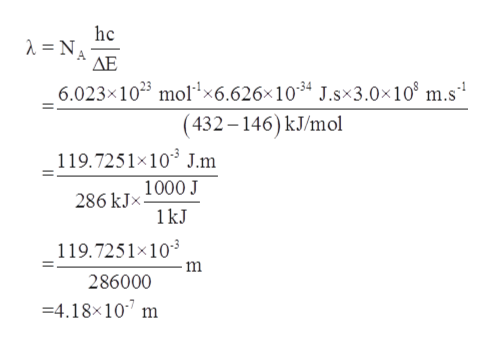# Be sure to answer all parts. The ionization energy of a certain element is 432 kJ/mol. (Ionization energy is the minimum energy required to remove an electron from an atom. It is usually expressed in units of kJ/mol; that is, it is the energy in kilojoules required to remove one mole of electrons from one mole of atoms.) However, when the atoms of this element are in the first excited state, the ionization energy is only 146 kJ/mol. Based on this information, calculate the wavelength of light emitted in a transition from the first excited state to the ground state. (Enter your answer in scientific notation.)

Question
503 views

Be sure to answer all parts.

The ionization energy of a certain element is 432 kJ/mol. (Ionization energy is the minimum energy required to remove an electron from an atom. It is usually expressed in units of kJ/mol; that is, it is the energy in kilojoules required to remove one mole of electrons from one mole of atoms.) However, when the atoms of this element are in the first excited state, the ionization energy is only 146 kJ/mol.

Based on this information, calculate the wavelength of light emitted in a transition from the first excited state to the ground state.

check_circle

Step 1

Given information:

Ionization energy of certain element = 432 kJ/mol

Ionization energy of that element for first excited state = 146 kJ/mol

Step 2

The expression for 1 photon of energy can be written in terms of Planck’s constant (h), speed of light (c)and wavelength (λ) as follows:

Step 3

The wavelength of light emitted in a transition from the first exci...help_outlineImage Transcriptionclosehc =NA ΔΕ 6.023x1023 mol1x6.626x1034 J.sx3.0x10 m.s1 (432-146) kJ/mol 119.7251 10 J.m 1000 J 286 kJx 1kJ 119.7251x103 286000 4.18x10 m fullscreen

### Want to see the full answer?

See Solution

#### Want to see this answer and more?

Solutions are written by subject experts who are available 24/7. Questions are typically answered within 1 hour.*

See Solution
*Response times may vary by subject and question.
Tagged in

### Atomic structure